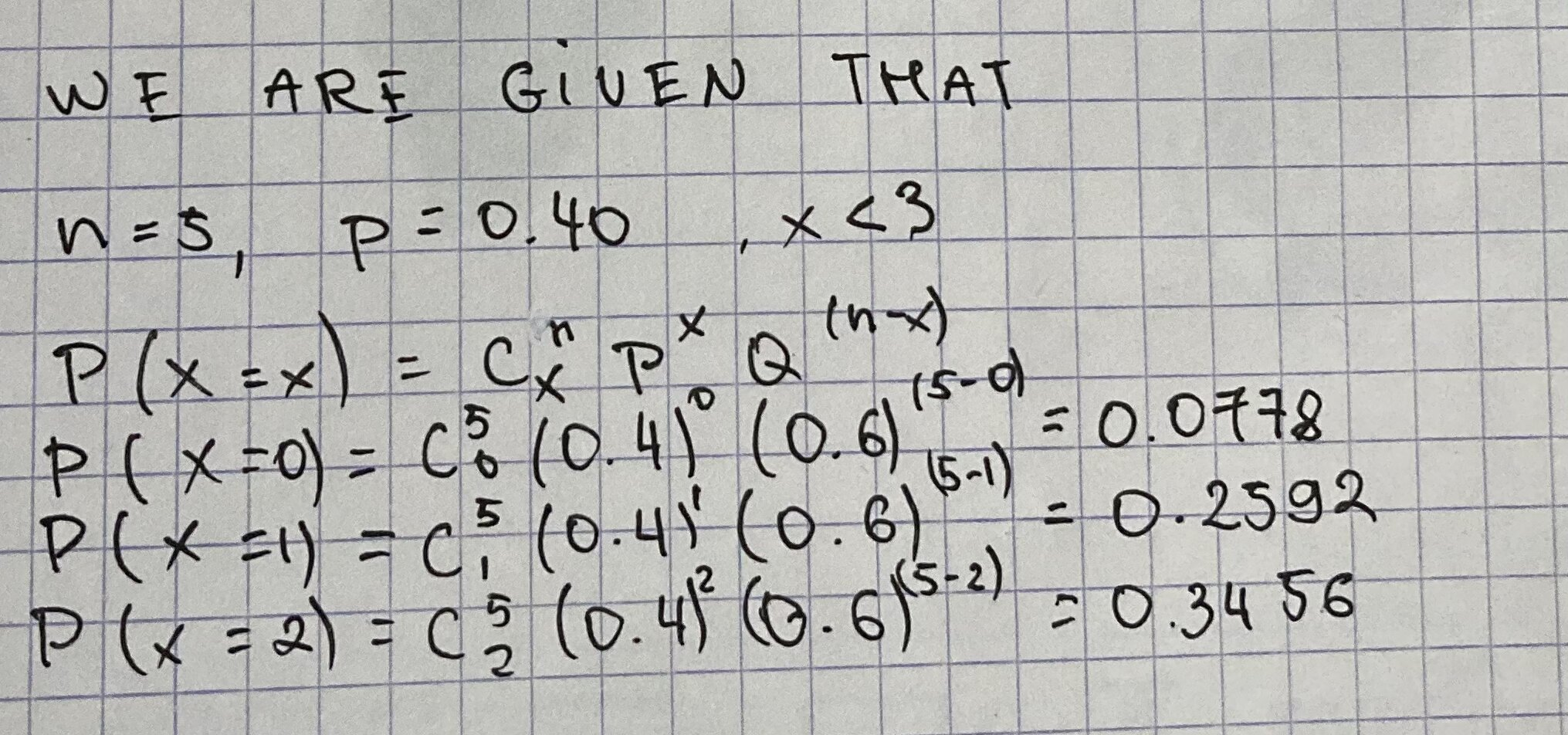# Question# If n=5 and p=0.40, what is the binomial probability that X < 3?

Binomial probability
ANSWEREDIf n=5 and p=0.40, what is the binomial probability that X < 3?